# reflectorCircular

Create circular reflector-backed antenna

## Description

Use the `reflectorCircular` object to create a circular reflector-backed antenna. By default the exciter is a dipole. The dimensions are chosen for an operating frequency of 1 GHz.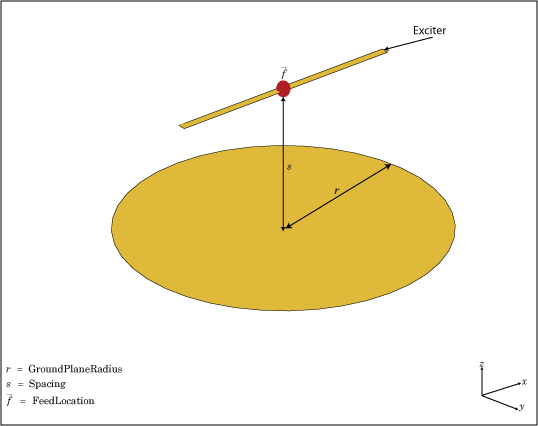## Creation

### Syntax

``rc = reflectorCircular``
``rc = reflectorCircular(Name=Value)``

### Description

example

````rc = reflectorCircular` creates a circular reflector backed antenna. ```

example

````rc = reflectorCircular(Name=Value)` creates a circular reflector antenna, with additional Properties specified by one or more name–value arguments. `Name` is the property name and `Value` is the corresponding value. You can specify several name-value arguments in any order as `Name1`= `Value1`, `...`, `NameN`=`ValueN`. Properties not specified retain their default values.For example, `rc = reflectorCircular(Radius=0.2)` creates a circular reflector of radius 0.2 m.```

## Properties

expand all

Exciter antenna or array type, specified as a single-element antenna object, an array object, or an empty array. Except for reflector and cavity antenna elements, you can use any Antenna Toolbox™ antenna or array element as an exciter. To create the cavity backing structure without the exciter, specify this property as an empty array.

Example: `horn`

Example: `linearArray(Element=patchMicrostrip)`

Example: `[]`

Radius of reflector, specified as a scalar in meters.

Example: `0.2`

Data Types: `double`

Distance between the exciter and the reflector, specified as a scalar in meters.

Example: `7.5e-2`

Data Types: `double`

Substrate dielectric material, specified as "air" or a `dielectric` object. For more information see, `dielectric`. For more information about dielectric substrate meshing, see Meshing.

Note

The substrate dimensions must be equal to the ground plane dimensions.

Example: `dielectric("FR4")`

Create probe feed from backing structure to exciter, specified as `0` (disable) or `1` (enable). By default, probe feed is disabled.

Example: `1`

Data Types: `double` | `logical`

Type of the metal used as a conductor, specified as a metal material object. You can choose any metal from the `MetalCatalog` or specify a metal of your choice. For more information, see `metal`. For more information on metal conductor meshing, see Meshing.

Example: `metal("Copper")`

Lumped elements added to the antenna feed, specified as a lumped element object. You can add a load anywhere on the surface of the antenna. By default, the load is at the origin. For more information, see `lumpedElement`.

Example: `Load=lumpedelement`. `lumpedelement` is the object for the load created using `lumpedElement`.

Example: `lumpedElement(Impedance=75)`

Tilt angle of the antenna in degrees, specified as a scalar or vector. For more information, see Rotate Antennas and Arrays.

Example: `90`

Example: `Tilt=[90 90]`,`TiltAxis=[0 1 0;0 1 1]` tilts the antenna at 90 degrees about the two axes defined by the vectors.

Data Types: `double`

Tilt axis of the antenna, specified as one of these values:

• Three-element vector of Cartesian coordinates in meters. In this case, each coordinate in the vector starts at the origin and lies along the specified points on the x-, y-, and z-axes.

• Two points in space, specified as a 2-by-3 matrix corresponding to two three-element vectors of Cartesian coordinates. In this case, the antenna rotates around the line joining the two points.

• `"x"`, `"y"`, or `"z"` to describe a rotation about the x-, y-, or z-axis, respectively.

Example: `[0 1 0]`

Example: `[0 0 0;0 1 0]`

Example: `"Z"`

Data Types: `double` | `string`

## Object Functions

 `show` Display antenna, array structures, shapes, or platform `axialRatio` Axial ratio of antenna `beamwidth` Beamwidth of antenna `charge` Charge distribution on antenna or array surface `current` Current distribution on antenna or array surface `design` Design prototype antenna or arrays for resonance around specified frequency or create AI-based antenna from antenna catalog objects `efficiency` Radiation efficiency of antenna `EHfields` Electric and magnetic fields of antennas or embedded electric and magnetic fields of antenna element in arrays `impedance` Input impedance of antenna or scan impedance of array `mesh` Mesh properties of metal, dielectric antenna, or array structure `meshconfig` Change meshing mode of antenna, array, custom antenna, custom array, or custom geometry `optimize` Optimize antenna or array using SADEA optimizer `pattern` Plot radiation pattern and phase of antenna or array or embedded pattern of antenna element in array `patternAzimuth` Azimuth plane radiation pattern of antenna or array `patternElevation` Elevation plane radiation pattern of antenna or array `rcs` Calculate and plot radar cross section (RCS) of platform, antenna, or array `returnLoss` Return loss of antenna or scan return loss of array `sparameters` Calculate S-parameters for antennas and antenna arrays `vswr` Voltage standing wave ratio (VSWR) of antenna or array element

## Examples

collapse all

Create and view a default circular reflector backed antenna.

`rc = reflectorCircular`
```rc = reflectorCircular with properties: Exciter: [1x1 dipole] Substrate: [1x1 dielectric] GroundPlaneRadius: 0.1000 Spacing: 0.0750 EnableProbeFeed: 0 Conductor: [1x1 metal] Tilt: 0 TiltAxis: [1 0 0] Load: [1x1 lumpedElement] ```
`show(rc)`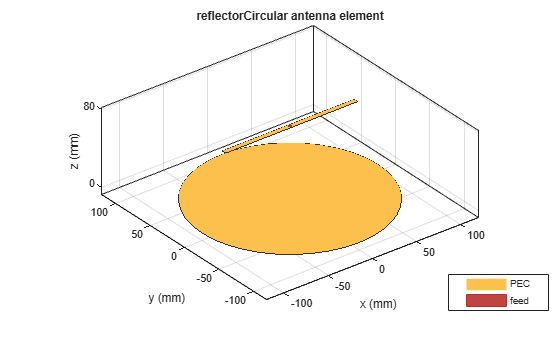Create an equiangular spiral backed by a circular reflector.

```ant = reflectorCircular(Exciter=spiralEquiangular,GroundPlaneRadius=0.02,... Spacing=0.01); show(ant)```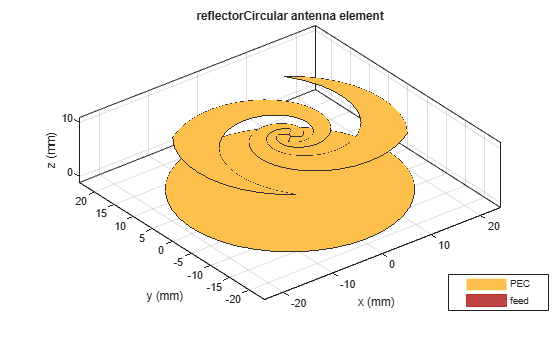Plot the radiation pattern of the antenna at 4 GHz.

`pattern(ant,4e9)`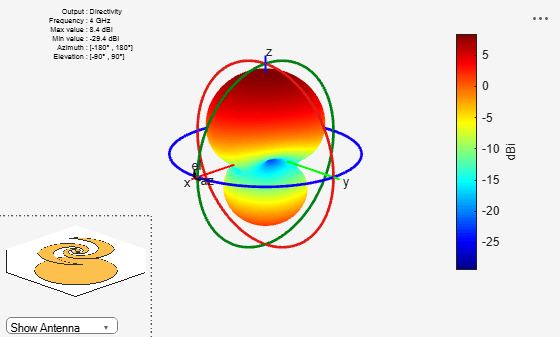Create a linear array of the dipole antennas.

```d = dipole(Length=1); la = linearArray(Element=d,NumElements=4,ElementSpacing=0.2,Tilt=90);```

Create a linear array with circular reflector backing structure.

`ant = reflectorCircular(Exciter=la,GroundPlaneRadius=2,Spacing=0.5)`
```ant = reflectorCircular with properties: Exciter: [1x1 linearArray] Substrate: [1x1 dielectric] GroundPlaneRadius: 2 Spacing: 0.5000 EnableProbeFeed: 0 Conductor: [1x1 metal] Tilt: 0 TiltAxis: [1 0 0] Load: [1x1 lumpedElement] ```
`show(ant)`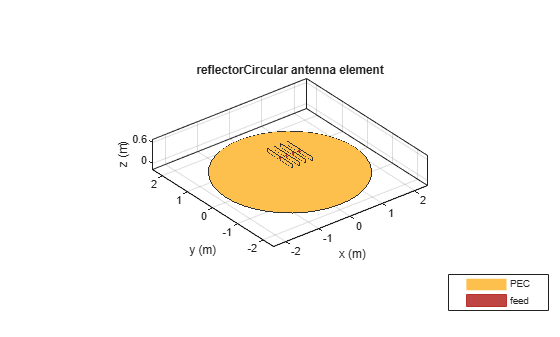Create a circular reflector-backed circular array of cylindrical DRAs.

```ca = circularArray(Element=draCylindrical,Radius=0.05); ant = reflectorCircular(Exciter=ca,GroundPlaneRadius=0.2)```
```ant = reflectorCircular with properties: Exciter: [1x1 circularArray] Substrate: [1x1 dielectric] GroundPlaneRadius: 0.2000 Spacing: 0.0750 EnableProbeFeed: 0 Conductor: [1x1 metal] Tilt: 0 TiltAxis: [1 0 0] Load: [1x1 lumpedElement] ```
`show(ant)`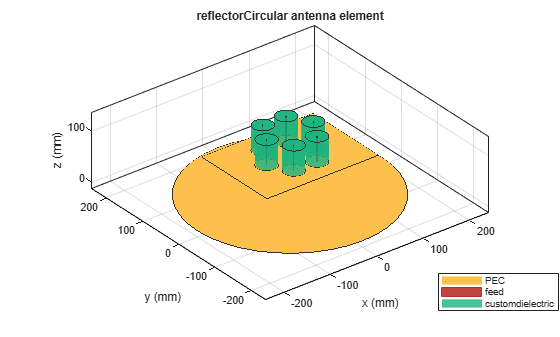## Version History

Introduced in R2017b